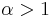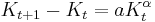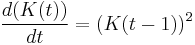# Long-run economic growth model

## Definition

The term long-run economic growth model is used for a model that describes how an economy grows in the long run. This growth may be measured via growth in measures such as gross domestic product, or via growth in total values of assets (land, capital, human capital). They may also be used to make long-run forecasts about the growth of specific economies, but this need not be their primary purpose.

Long-run economic growth models differ from each other in terms of the extent to which they account for, and the extent to which they endogenize, population growth, human capital improvements, accumulation of physical capital, and technological progress. They also differ in terms of the level and nature of their microfoundations.

Long-run economic growth models differ from short-run models that generally focus more on the role of fiscal and monetary policy, animal spirits, and regime uncertainty.

## Stylized basic model

• Inputs (such as labor, capital, and technology) that could vary from year to year, determine outputs at a given point of time (or in a given year), via a fixed functional relationship (called a production function). The production function itself is fixed.
• The inputs themselves can change from year to year. Part of this change is through feedback from the output: some of the output gets allocated to investment such as investment in physical capital, investment in human capital (either through population growth or through improving the skill level of the existing population), or investment in technological improvement. The extent to which this happens depends on the economy's savings rate. However, there could also be changes to inputs arising from other factors (such as serendipitious discovery of new technology, or discovery of new natural resources, or other "random chance" factors).

## Continuous and discrete models

### Basic distinction

Discrete models use fixed time intervals, such as years. In a given year, the output is determined from the inputs via a production function. The part of the output that gets saved then determines changes to the inputs for next year, which in turn determine the output for that year, and so on. To "solve" this type of model given some initial conditions, we simply compute the inputs and outputs for successive years one at a time.

In continuous models, the output per unit time is computed using the production function of the input per unit time. Further, the rate of change of inputs with respect to time (formally given as the derivative of the inputs with respect to time) is expressed in terms of the current values of inputs and outputs. We thus obtain a differential equation. We can then solve this differential equation using mathematical techniques and thereby get a picture of how the economy grows over time.

### Caveat about converting a discrete model to the analogous continuous model

Naively, one might expect that the discrete model:

(Change in input from one year to the next) = (Some expression$F$ involving inputs and outputs)

and the continuous model:

(Rate of change in input from one year to the next) = (The same expression$F$ involving inputs and outputs)

lead to the same qualitative predictions. And this is true when$F$ is relatively "small" but fails when$F$ grows fast. In the latter case, continuous models predict much faster growth rate than discrete models. The reason, roughly, is that continuous models allow for very rapid feedback from inputs to outputs, that escalates rather quickly, whereas discrete models enforce a year's time lag regardless of how quickly the inputs and outputs are growing. In particular, consider a model of the form:$\frac{dK}{dt} = aK^\alpha$

where$\alpha > 1$. This has an asymptote at finite time. On the other hand, the discrete version:$K_{t+1} - K_t = aK_t^\alpha$

grows superexponentially, but does not reach infinity in finite time.

### Delay differential equations: combining the lagged nature of discrete models with the infinite divisibility of continuous models

A delay differential equation is a variant of the concept of differential equation where one is allowed to use the value of a variable a fixed amount of time in the past. In the context of the economic growth model, a delay differential equation might be something like:$\frac{d(K(t))}{dt} = (K(t - 1))^2$

This says that the rate of growth of the capital stock is proportional to the square of the capital stock one time unit ago (i.e., one year ago, if time is measured in years) ago. Delay differential equations therefore capture the lagged nature of discrete models while still allowing for continuous change.

## What the model accounts for

Models differ in the manner and extent to which they account for particular aspect. Here are some possibilities:

• The model doesn't account for the aspect explicitly, instead subsuming it in general constants.
• The model accounts for the aspect, but treats it as a constant for a given economy (still allowing for different values for different economies).
• The model accounts for the aspect, but treats it as having an explicitly defined growth rate (based on some off-model empirical analysis) without explaining theoretically how that growth rate was observed.
• The model accounts for the aspect, but treats the value each year as something that is provided from outside the model (from empirical data).
• The model accounts for the aspect and includes a story for how that aspect changes, so the story is part of the model. The story may involve some sort of optimization for the present or the future.

When we say that a model endogenizes a particular aspect, we usually mean the last of these: the model has a story for how that aspect changes, and the story involves some sort of optimization for the present or the future.